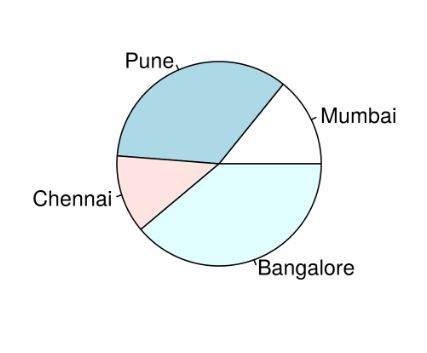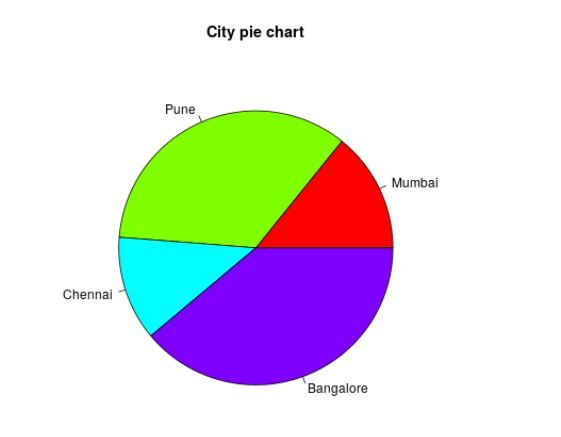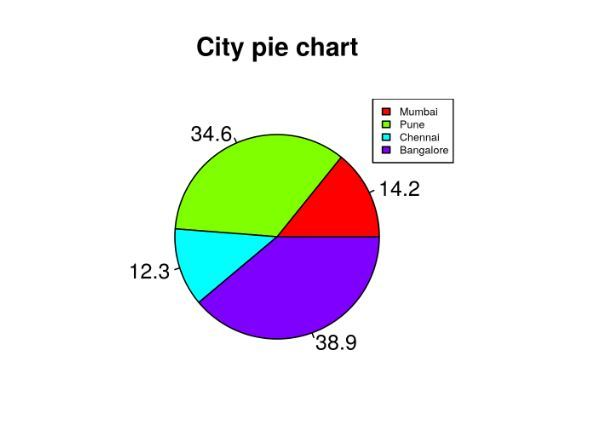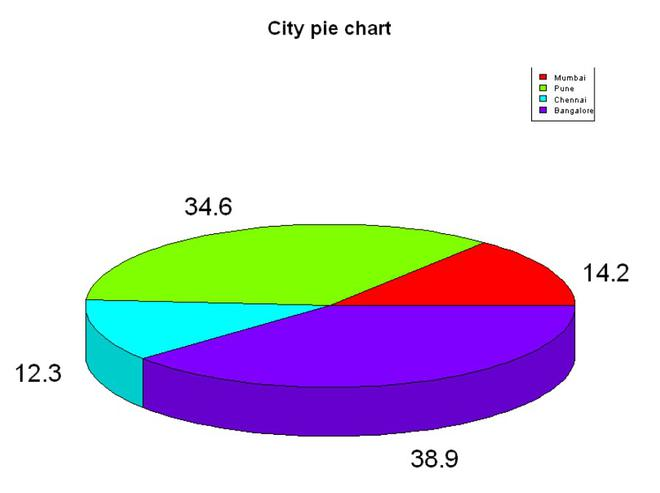# R – Pie Charts

• Last Updated : 09 Dec, 2021

A pie chart is a circular statistical graphic, which is divided into slices to illustrate numerical proportions. It depicts a special chart that uses “pie slices”, where each sector shows the relative sizes of data. A circular chart cuts in a form of radii into segments describing relative frequencies or magnitude also known as a circle graph.

## R – Pie Charts

R Programming Language uses the function pie() to create pie charts. It takes positive numbers as a vector input.

Syntax: pie(x, labels, radius, main, col, clockwise)

Parameters:

• x: This parameter is a vector that contains the numeric values which are used in the pie chart.
• labels: This parameter gives the description to the slices in pie chart.
• radius: This parameter is used to indicate the radius of the circle of the pie chart.(value between -1 and +1).
• main: This parameter is represents title of the pie chart.
• clockwise: This parameter contains the logical value which indicates whether the slices are drawn clockwise or in anti clockwise direction.
• col: This parameter give colors to the pie in the graph.

### Creating a simple pie chart

To create a simple pie chart:

• By using the above parameters, we can draw a pie chart.
• It can be described by giving simple labels.

Example:

## R

 `# Create data for the graph.``geeks<- ``c``(23, 56, 20, 63)``labels <- ``c``(``"Mumbai"``, ``"Pune"``, ``"Chennai"``, ``"Bangalore"``)` `# Plot the chart.``pie``(geeks, labels)`

Output:### Pie chart including the title and colors

To create color and title pie chart.

• Take all parameters which are required to make pie chart by giving a title to the chart and add labels.
• We can add more features by adding more parameters with more colors to the points.

Example:

## R

 `# Create data for the graph.``geeks<- ``c``(23, 56, 20, 63)``labels <- ``c``(``"Mumbai"``, ``"Pune"``, ``"Chennai"``, ``"Bangalore"``)` `# Plot the chart with title and rainbow``# color pallet.``pie``(geeks, labels, main = ``"City pie chart"``,``            ``col = ``rainbow``(``length``(geeks)))`

Output:### Slice Percentage & Chart Legend

To create chart legend and slice percentage, we can plot by doing the below methods.

• There are two more properties of the pie chart:
• slice percentage
• chart legend.
• We can show the chart in the form of percentages as well as add legends.

Example:

## R

 `# Create data for the graph.``geeks <- ``c``(23, 56, 20, 63)``labels <- ``c``(``"Mumbai"``, ``"Pune"``, ``"Chennai"``, ``"Bangalore"``)` `piepercent<- ``round``(100 * geeks / ``sum``(geeks), 1)` `# Plot the chart.``pie``(geeks, labels = piepercent,``    ``main = ``"City pie chart"``, col = ``rainbow``(``length``(geeks)))``legend``(``"topright"``, ``c``(``"Mumbai"``, ``"Pune"``, ``"Chennai"``, ``"Bangalore"``),``                    ``cex = 0.5, fill = ``rainbow``(``length``(geeks)))`

Output:## 3D Pie Chart

Here we are going to create a 3D Pie chart using plotrix package and then we will use pie3D() function to plot 3D plot.

## R

 `# Get the library.``library``(plotrix)` `# Create data for the graph.``geeks <- ``c``(23, 56, 20, 63)``labels <- ``c``(``"Mumbai"``, ``"Pune"``, ``"Chennai"``, ``"Bangalore"``)` `piepercent<- ``round``(100 * geeks / ``sum``(geeks), 1)` `# Plot the chart.``pie3D``(geeks, labels = piepercent,``    ``main = ``"City pie chart"``, col = ``rainbow``(``length``(geeks)))``legend``(``"topright"``, ``c``(``"Mumbai"``, ``"Pune"``, ``"Chennai"``, ``"Bangalore"``),``                    ``cex = 0.5, fill = ``rainbow``(``length``(geeks)))`

Output:My Personal Notes arrow_drop_up